﻿ Influence and Strategy of Electric Vehicle Charging on Distribution Network Voltage Based on Uncertainty Charging HabitPublications are Open
Access in this journal
Article Versions
Export Article
• Normal Style
• MLA Style
• APA Style
• Chicago Style
Research Article
Open Access Peer-reviewed

### Influence and Strategy of Electric Vehicle Charging on Distribution Network Voltage Based on Uncertainty Charging Habit

Lu Mengtian
Journal of Automation and Control. 2017, 5(1), 20-24. DOI: 10.12691/automation-5-1-4
Published online: July 04, 2017

### Abstract

With the increasing scale of the use of electric vehicles, impact of electric vehicle charging on the distribution system cannot be ignored. Considering uncertainty in the charging behavior, large-scale disorderly charging will cause the voltage deviation of distribution network exceeds the specified value. In the paper, based on the IEEE33 node as an example, the goal is to minimum each node voltage deviation by to controlling charge power, considering the constraints of the charging energy and the charging time, and optimized by the genetic algorithm. By using Newton Raphson power flow method, the voltage offset of each node before and after optimization is obtained, and the effectiveness of the charging load optimization model is verified by comparing and analyzing.

### 1. Introduction

In the context of worsening environmental conditions, fossil fuels are tight, in order to reduce the emissions of greenhouse gas, the electric car as the green traffic tool is used to replace the traditional fuel vehicles which has been vigorously promoted and developed rapidly. There are many researches on the performance optimization of EV and further reduction of air pollution 1 and some researches on how to make a progress in fuel economy for various driving patterns 2, 3. It is foreseeable that in the near future, there will be large-scale electric vehicles connected to the grid for charging. The users’ charging behavior is uncertain, so if their charging behavior is not controlled, the large-scale electric vehicles’ charging will have an impact on the distribution network 4. Therefore, it is necessary to study and predict the impact of the electric vehicle charging, which is of great significance to the operation and construction of the distribution network.

Until now, some research has studied the impacts of electric vehicle charging on distribution network, mainly for electric vehicle charging load influence on distribution network loss; charging load impact on system load 5, 6. However, for the medium and low voltage power network, few research studies the voltage offset and harmonics problems of the distribution network caused by electric vehicle charging. Secondly, the most studies mainly consider the orderly charging of electric vehicle model, only a small part take into account the uncertainty of the charging habits, even if some research 7 think about the uncertain charging habits but only considered the charging load and optimization and did not consider the impact on the voltage offset of the distribution network under the model. Therefore, this paper mainly studies the voltage offset of the distribution network at different permeability when electric vehicles are charging under the charging model of the uncertain charging habit.

The research object of this paper is the offset voltage of the node, if the user in charge of charging pile, the charging pile is of rational planning, each node is relatively evenly distributed, the topology of distribution network is radial and the root node connects outside the grid, the paper study the root node.

### 2. EV Charging Load Model and Method of Charging Demand Calculation

2.1. Model of Uncertainty Charging Habits

If we want to study the charging situation of electric vehicles, we should understand the charging time and charging power, but think about highly subjective user groups of electric vehicles, the charging situation is uncertain. For individual user, the charge status can be divided into two kinds, namely "charging" and "stop charging", "charging" is expressed in "1", "stop charging" is expressed in "0", so the user group charge state obeys (0-1) distribution. Secondly, when the user is in the "charging" state, the situation is also divided into two categories, namely "fast charging" and "conventional charging", and respectively expressed in "0" and "1" which also obeys (0-1) distribution. In addition to the user's subjective impact on the charging situation, a number of external factors will affect the user's charging situation, for example, users charging behavior will reduce when electricity prices are expensive or increase when cheap, so at the same time, the number of electric cars connected to the grid is negatively correlated with the price at that time. Due to the operation of Chinese electric vehicles has not yet reached the large-scale, so this paper adopts the method adopted by most research which through the study of the traditional fuel vehicle travel rules, assumes that the electric vehicle travel law is basically the same and calculate charging demand.

2.2. Calculation Method of Charging Demand

(1) The group of EV includes public traffic, transport traffic and private vehicles. Considering the charging time of public traffic is relative fixation and of private vehicles is uncertain, besides, the private EV constitutes the main group of electric vehicles ,so the paper build the charging model which only takes the private cars into consideration. The paper uses Monte Carlo simulation method to calculate charging demand 8. The rand function generates a random number x on [0,1] in MATLAB and according to the travel rules of traditional fuel vehicles to calculate the probability of each travel time, by comparing the random number x and the probability distribution to determine the time period for the generated random number. In most studies, the paper assumes that the use of electric vehicles does not change the existing travel rules. Considering the charging behavior of electric car users will be affected by the price points, so the paper assumes that the charge behavior will be appropriately reduced in peak price period or increased in low price period and in other periods of charging behavior follow the rules of the traditional fuel vehicles travel. The EV charging situations of different driving conditions are shown in the Table 1:

(2) We can get the SOC probability distribution according to the charging period produced in the first step and then using the corresponding function in MATLAB to get the initial charge status of the electric car. Assuming that the electric vehicle charging power is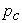and the total capacity of the electric car is S, the situation of the charge of the battery before and after the electric car is charged is shown in the form (1):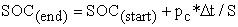(1)

In the formula,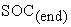,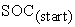respectively respect charge state of the battery before and after charging.

(3) Simulating the situation of N electric vehicles by MATLAB and finally load the charging load of N electric vehicle to get the total charging load at each moment, such as formula (2):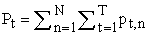(2)

(4) Objective function

This paper aims at minimizing the sum of voltage offset per node, as shown in equation (3):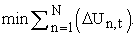(3)

In this paper, the day 24h is divided into 96 periods of time on average, each time period is 15min, the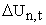said the t time the voltage offset of the nth node, N said the total number of nodes, in this article, N is 33.

(5) Constraints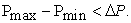(4)

Formula (4) is the constraint formula for difference of peak and valley.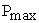and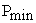respectively indicates the maximum and minimum values of the load within a day 24h. The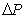indicates the minimum value of the difference between peak and valley in the past week. Thewill increase by 1% when the optimization model has no solution, until we have a solution.

The paper simulated the charging load curve of EV according to the charging model mentioned above in MATLAB. In the paper, BTD E6 is taken as an example. SOC will have some impact on the HEV's driving, but no impact on the electric vehicles which belong to BEV and BYD E6 belongs to the latter, so this article does not discuss the impact of SOC on EV traffic. The simulation parameters are as follows: Battery capacity is 57(Kwh), uumber of kilometers per unit of pure electric travel is 316(Km), charging duration is 7(h), the power of charging is 6.3(Kw/h). The simulation results are shown in Figure 1:

• Figure 1. Original curve of charging load
2.3. Impact of Electric Vehicle Charging on the Voltage

In order to study the impact of EV charging load on voltage of distribution network, this paper uses the Newton Raphson method to calculate the flow of the system so we can get the voltage of each node under different permeability and finally get the voltage offset of each node by processing the data, as shown in Figure 2. We can find that with the increase of permeability, the voltage offset is also increased significantly. According to the quality of national power, the allowable deviation is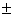7% of the rated voltage for 10kv and the following three-phase power supply voltage. When the permeability is at 5% and below, the voltage offset at the end of the line is about 6%, which can basically guarantee the power quality of the charging user. When permeability rises to 10%, the node voltage deviation starts out-of-limit. When the permeability continue to raise to 30%, the end node voltage deviation increases rapidly and the maximum value is of 8.4% around which is already serious out-of-limit.

• Figure 2. Node voltage offset

Table 2 shows the situation of each node under the different permeability. When the permeability is less than or equal to 5%, there is no node out-of-limit. With the gradual increase of permeability, the nodes out-of-limit also increased rapidly and the maximum value of voltage offset increases. When the permeability is 30%, the proportion of the nodes out-of-limit is as high as 24.4% and the maximum deviation rate is as high as 8.4%, has obviously exceeded the national standard of 7%.

2.4. Genetic Algorithm

In order to optimize the model, people put forward the hill-climbing algorithm, particle swarm algorithm and genetic algorithm optimization method. Method of climbing is high precision but easy to fall into local minima. The swarm has high convergence speed but may fall into local optimum. Genetic algorithm is based on the biological nature of the survival of the fittest and the method through selection, crossover and mutation operation to search the optimal solution in the problem space. However, the classic genetic algorithm also has the problem of falling into local minima. To sum up, in order to prevent the problem mentioned above, this paper used the improved genetic algorithm to optimize the model, which can enhance the global optimization, and the optimization speed.

The specific steps are as follows: The first step is to initialize groups; The second step is to compute the fitness values of each individual of the swarm; The third step is to select the individuals that will enter the next generation according to the rules determined by the individual fitness value; The fourth step is to perform crossover operation according to probability cp; The fifth step is to perform mutation operation according to probability mp; The sixth step is to performs the seventh step if algorithm meets the stop conditions, otherwise to perform the second step. There are 3 stop conditions of the algorithm. The first is to complete the pre-given evolutionary algebra; the second is the best individual or the average fitness have no improvement in successive generations; the third is the optimal value is less than the given threshold. The seventh step is to produce the chromosome with the best fitness value in the population as the satisfactory solution of the problem.

### 3. Example Analysis

3.1. Network Model

The IEEE33 node is selected to investigate the impact of electric vehicles charging on voltage of the distribution network and its topology is shown in Figure 3, in this network, there are 32 branches, 33 nodes, a reference voltage power network is 12.66KV, reference value of three-phase power is 10MVA, the total power load of network is 5084.26KW, the power factor of system is 0.95. The node 1 is the equilibrium node, and the nominal voltage of the remaining nodes is 1.

• Figure 3. Topology of system network distribution IEEE33 node
3.2. Specific Settings of GA Parameters

There are 250 individuals in the population, the total number of genetic iterations is 90, the maximum probability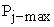is 0.85, the maximum variation probability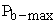is 0.1, the minimum probability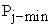is 0.45 and the minimum variation probabilityis 0.01.

3.3. Optimization Results

Firstly, in MATLAB, the IEEE33 node is calculated by Newton Raphson method according to the known data: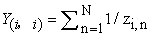(5)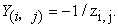(6)

Formula (5) and formula (6) are admittance matrix formulas,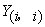is the sum of the bottom of the reciprocal impedances between all nodes connected to the i node, and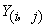is the opposite of the reciprocal between the node i and the node impedance.

(2) Generate Jacobi matrix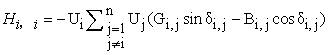(7)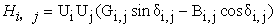(8)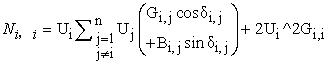(9)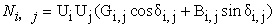(10)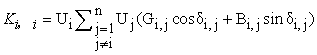(11)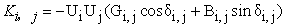(12)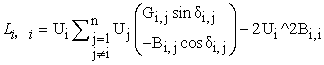(13)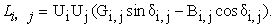(14)

Formula (7) to (14) are formulas for calculating the four submatrices in Jacobi matrix,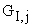,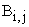is the conductance and susceptance between node i and node j.

(3) Correction is obtained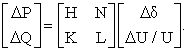(15)

By type (15), the amount of the correction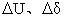can be obtained. Through iteration, when precision meets the convergence criteria, calculation ends.

• Figure 4. Figure before and after optimization
• Figure 5. Optimized curve of charging load

Figure 4 shows the optimization results. Take the permeability of 20% as an example and get the voltage offset curve of each node of IEEE33 before and after optimization. We can find that there are 12.12% of nodes out-of-limits and the maximum voltage deviation rate is about 7.5%, has exceeded the national standard, through the optimization, the maximum offset voltage rate has dropped to 6.8%, all nodes are within the scope of the standard, the voltage deviation of each node has decreased significantly after 11 nodes, decreased 0.48% on average, the voltage deviation decreased 0.74% at 33 node. Figure 5 shows the optimized load curve. We can find that the valley occurs at around 4 a.m. and the peak value occurs at around 21 p.m. Compared with the original curve of charging load, the charging load has been improved at valley and has been reduced at peak, so the peak-to-trough has been reduced and the load curve becomes smoother. The optimization has an ideal effect.

### 4. Conclusion

This paper presents an optimization model and solving method of control strategy of electric vehicle charging based on charging habits with uncertainty, the model considers the constraint of difference between the peak and valley of charging load, and take minimizing the per node voltage offset as the goal, and simulating the IEEE33 system, using Newton Raphson method to perform power flow calculation with known data, through the collation and analysis of data, obtained in different permeability, voltage offset of each node of the IEEE33 system, this paper uses genetic algorithm to optimize the model, and contrast the voltage offset before and after simulation in 20% permeability and verify the effectiveness of this model and method.

### Acknowledgements

This work was conducted partly at Nanjing normal university Research Lab. Thanks are due to Prof. Ma Gang for his support.

### References

  Morteza, Montazeri-Gh and Mehdi Mahmoodi-k, “Development a new power management strategy for power split hybrid electric vehicles”, Transportation Research Part D: Transport and Environment, vol. 37, no.7, pp.79-96, 2015. In article View Article  Morteza Montazeri-Gh and Mehdi Mahmoodi-K, “Optimized predictive energy management of plug-in hybrid electric vehicle based on traffic condition”, Journal of Cleaner Production, vol. 139, no. 15, pp. 935-948, 2016. In article View Article  MK Dayeni, M Soleymani and AA Ghadimi, “Designing an optimal fuzzy controller for a fuel cell vehicle conside ring drivingpatterns”, Scientia Iranica, 2015. In article View Article  WANG Hui, WEN fu-shuan, XIN Jian-bo, Charging and discharging characteristics of electric vehicles as well as their impacts 0n distribution systems. Journal of Noah China Electric Power University, 2011, 38(5). In article View Article  LI HUI-lin, BAI XIAO-min, Influence of electric vehicle charging on distribution network and Countermeasures. Automation of Electric Power Systems, 2011, 35(17). In article  GUO Jian-long, WEN Fu-shuan. The influence of electric vehicle charging on power system and Its Countermeasures. Electric Power Automation Equipment, 2015, 35(6). In article  Yang Bing, Wang Lifang, Liao Chenglin, Ji Li, Dong Fengyu, Effects of Uncertainty Charging Habits on Electric Vehicles’ Charging Load Demand and the Charging Load Regulation. TRANSACTIONS OF CHINA ELECTROTECHNICAL SOCIETY, 2015, 30(4). In article  PANG Pei-chuan, ZENG Cheng, YANG Biao, ZHANG Qian, Calculation of Electric Vehicles Charging Load by Monte Carlo Simulation Method. Telecom Power Technology, 2016, 33(1). In article  FENG Chao, ZHOU Bu-xian, LIN Nan, XU Fei, LI Yan91, XIA Yu-han91, Electric Vehicle Charging Station Planning Based on Multiple-population Hybrid Genetic Algorithm, Proceedings of the CSU-EPSA, 2013, 25(6). In article View Article  ZHANG Cong, XU Xiao-hui, SUN Hai-shun, ZHOU Xin. Smart charging strategy of large-scale electric vehicles based on adaptive genetic algorithm. Power System Protection and Control, 2014, 42(14). In articleThis work is licensed under a Creative Commons Attribution 4.0 International License. To view a copy of this license, visit http://creativecommons.org/licenses/by/4.0/